Professor Murray

Inverse Trigonometric Functions

Slide Duration:

Section 1: Trigonometric Functions
Angles

39m 5s

Intro
0:00
Degrees
0:22
Circle is 360 Degrees
0:48
Splitting a Circle
1:13
2:08
2:31
2:52
Half-Circle and Right Angle
4:00
6:24
6:52
Coterminal, Complementary, Supplementary Angles
7:23
Coterminal Angles
7:30
Complementary Angles
9:40
Supplementary Angles
10:08
Example 1: Dividing a Circle
10:38
Example 2: Converting Between Degrees and Radians
11:56
Example 3: Quadrants and Coterminal Angles
14:18
Extra Example 1: Common Angle Conversions
-1
Extra Example 2: Quadrants and Coterminal Angles
-2
Sine and Cosine Functions

43m 16s

Intro
0:00
Sine and Cosine
0:15
Unit Circle
0:22
Coordinates on Unit Circle
1:03
Right Triangles
1:52
2:25
Master Right Triangle Formula: SOHCAHTOA
2:48
Odd Functions, Even Functions
4:40
Example: Odd Function
4:56
Example: Even Function
7:30
Example 1: Sine and Cosine
10:27
Example 2: Graphing Sine and Cosine Functions
14:39
Example 3: Right Triangle
21:40
Example 4: Odd, Even, or Neither
26:01
Extra Example 1: Right Triangle
-1
Extra Example 2: Graphing Sine and Cosine Functions
-2
Sine and Cosine Values of Special Angles

33m 5s

Intro
0:00
45-45-90 Triangle and 30-60-90 Triangle
0:08
45-45-90 Triangle
0:21
30-60-90 Triangle
2:06
Mnemonic: All Students Take Calculus (ASTC)
5:21
Using the Unit Circle
5:59
New Angles
6:21
9:43
Mnemonic: All Students Take Calculus
10:13
13:11
16:48
Example 3: All Angles and Quadrants
20:21
Extra Example 1: Convert, Quadrant, Sine/Cosine
-1
Extra Example 2: All Angles and Quadrants
-2
Modified Sine Waves: Asin(Bx+C)+D and Acos(Bx+C)+D

52m 3s

Intro
0:00
Amplitude and Period of a Sine Wave
0:38
Sine Wave Graph
0:58
Amplitude: Distance from Middle to Peak
1:18
Peak: Distance from Peak to Peak
2:41
Phase Shift and Vertical Shift
4:13
Phase Shift: Distance Shifted Horizontally
4:16
Vertical Shift: Distance Shifted Vertically
6:48
Example 1: Amplitude/Period/Phase and Vertical Shift
8:04
Example 2: Amplitude/Period/Phase and Vertical Shift
17:39
Example 3: Find Sine Wave Given Attributes
25:23
Extra Example 1: Amplitude/Period/Phase and Vertical Shift
-1
Extra Example 2: Find Cosine Wave Given Attributes
-2
Tangent and Cotangent Functions

36m 4s

Intro
0:00
Tangent and Cotangent Definitions
0:21
Tangent Definition
0:25
Cotangent Definition
0:47
Master Formula: SOHCAHTOA
1:01
Mnemonic
1:16
Tangent and Cotangent Values
2:29
Remember Common Values of Sine and Cosine
2:46
90 Degrees Undefined
4:36
Slope and Menmonic: ASTC
5:47
Uses of Tangent
5:54
Example: Tangent of Angle is Slope
6:09
7:49
Example 1: Graph Tangent and Cotangent Functions
10:42
Example 2: Tangent and Cotangent of Angles
16:09
Example 3: Odd, Even, or Neither
18:56
Extra Example 1: Tangent and Cotangent of Angles
-1
Extra Example 2: Tangent and Cotangent of Angles
-2
Secant and Cosecant Functions

27m 18s

Intro
0:00
Secant and Cosecant Definitions
0:17
Secant Definition
0:18
Cosecant Definition
0:33
Example 1: Graph Secant Function
0:48
Example 2: Values of Secant and Cosecant
6:49
Example 3: Odd, Even, or Neither
12:49
Extra Example 1: Graph of Cosecant Function
-1
Extra Example 2: Values of Secant and Cosecant
-2
Inverse Trigonometric Functions

32m 58s

Intro
0:00
Arcsine Function
0:24
Restrictions between -1 and 1
0:43
Arcsine Notation
1:26
Arccosine Function
3:07
Restrictions between -1 and 1
3:36
Cosine Notation
3:53
Arctangent Function
4:30
Between -Pi/2 and Pi/2
4:44
Tangent Notation
5:02
Example 1: Domain/Range/Graph of Arcsine
5:45
Example 2: Arcsin/Arccos/Arctan Values
10:46
Example 3: Domain/Range/Graph of Arctangent
17:14
Extra Example 1: Domain/Range/Graph of Arccosine
-1
Extra Example 2: Arcsin/Arccos/Arctan Values
-2
Computations of Inverse Trigonometric Functions

31m 8s

Intro
0:00
Inverse Trigonometric Function Domains and Ranges
0:31
Arcsine
0:41
Arccosine
1:14
Arctangent
1:41
Example 1: Arcsines of Common Values
2:44
Example 2: Odd, Even, or Neither
5:57
Example 3: Arccosines of Common Values
12:24
Extra Example 1: Arctangents of Common Values
-1
Extra Example 2: Arcsin/Arccos/Arctan Values
-2
Section 2: Trigonometric Identities
Pythagorean Identity

19m 11s

Intro
0:00
Pythagorean Identity
0:17
Pythagorean Triangle
0:27
Pythagorean Identity
0:45
Example 1: Use Pythagorean Theorem to Prove Pythagorean Identity
1:14
Example 2: Find Angle Given Cosine and Quadrant
4:18
Example 3: Verify Trigonometric Identity
8:00
Extra Example 1: Use Pythagorean Identity to Prove Pythagorean Theorem
-1
Extra Example 2: Find Angle Given Cosine and Quadrant
-2
Identity Tan(squared)x+1=Sec(squared)x

23m 16s

Intro
0:00
Main Formulas
0:19
Companion to Pythagorean Identity
0:27
For Cotangents and Cosecants
0:52
How to Remember
0:58
Example 1: Prove the Identity
1:40
Example 2: Given Tan Find Sec
3:42
Example 3: Prove the Identity
7:45
Extra Example 1: Prove the Identity
-1
Extra Example 2: Given Sec Find Tan
-2

52m 52s

Intro
0:00
0:09
How to Remember
0:48
Cofunction Identities
1:31
How to Remember Graphically
1:44
Where to Use Cofunction Identities
2:52
Example 1: Derive the Formula for cos(A-B)
3:08
Example 2: Use Addition and Subtraction Formulas
16:03
Example 3: Use Addition and Subtraction Formulas to Prove Identity
25:11
Extra Example 1: Use cos(A-B) and Cofunction Identities
-1
Extra Example 2: Convert to Radians and use Formulas
-2
Double Angle Formulas

29m 5s

Intro
0:00
Main Formula
0:07
How to Remember from Addition Formula
0:18
Two Other Forms
1:35
Example 1: Find Sine and Cosine of Angle using Double Angle
3:16
Example 2: Prove Trigonometric Identity using Double Angle
9:37
Example 3: Use Addition and Subtraction Formulas
12:38
Extra Example 1: Find Sine and Cosine of Angle using Double Angle
-1
Extra Example 2: Prove Trigonometric Identity using Double Angle
-2
Half-Angle Formulas

43m 55s

Intro
0:00
Main Formulas
0:09
Confusing Part
0:34
Example 1: Find Sine and Cosine of Angle using Half-Angle
0:54
Example 2: Prove Trigonometric Identity using Half-Angle
11:51
Example 3: Prove the Half-Angle Formula for Tangents
18:39
Extra Example 1: Find Sine and Cosine of Angle using Half-Angle
-1
Extra Example 2: Prove Trigonometric Identity using Half-Angle
-2
Section 3: Applications of Trigonometry
Trigonometry in Right Angles

25m 43s

Intro
0:00
Master Formula for Right Angles
0:11
SOHCAHTOA
0:15
Only for Right Triangles
1:26
Example 1: Find All Angles in a Triangle
2:19
Example 2: Find Lengths of All Sides of Triangle
7:39
Example 3: Find All Angles in a Triangle
11:00
Extra Example 1: Find All Angles in a Triangle
-1
Extra Example 2: Find Lengths of All Sides of Triangle
-2
Law of Sines

56m 40s

Intro
0:00
Law of Sines Formula
0:18
SOHCAHTOA
0:27
Any Triangle
0:59
Graphical Representation
1:25
Solving Triangle Completely
2:37
When to Use Law of Sines
2:55
ASA, SAA, SSA, AAA
2:59
SAS, SSS for Law of Cosines
7:11
Example 1: How Many Triangles Satisfy Conditions, Solve Completely
8:44
Example 2: How Many Triangles Satisfy Conditions, Solve Completely
15:30
Example 3: How Many Triangles Satisfy Conditions, Solve Completely
28:32
Extra Example 1: How Many Triangles Satisfy Conditions, Solve Completely
-1
Extra Example 2: How Many Triangles Satisfy Conditions, Solve Completely
-2
Law of Cosines

49m 5s

Intro
0:00
Law of Cosines Formula
0:23
Graphical Representation
0:34
Relates Sides to Angles
1:00
Any Triangle
1:20
Generalization of Pythagorean Theorem
1:32
When to Use Law of Cosines
2:26
SAS, SSS
2:30
Heron's Formula
4:49
Semiperimeter S
5:11
Example 1: How Many Triangles Satisfy Conditions, Solve Completely
5:53
Example 2: How Many Triangles Satisfy Conditions, Solve Completely
15:19
Example 3: Find Area of a Triangle Given All Side Lengths
26:33
Extra Example 1: How Many Triangles Satisfy Conditions, Solve Completely
-1
Extra Example 2: Length of Third Side and Area of Triangle
-2
Finding the Area of a Triangle

27m 37s

Intro
0:00
Master Right Triangle Formula and Law of Cosines
0:19
SOHCAHTOA
0:27
Law of Cosines
1:23
Heron's Formula
2:22
Semiperimeter S
2:37
Example 1: Area of Triangle with Two Sides and One Angle
3:12
Example 2: Area of Triangle with Three Sides
6:11
Example 3: Area of Triangle with Three Sides, No Heron's Formula
8:50
Extra Example 1: Area of Triangle with Two Sides and One Angle
-1
Extra Example 2: Area of Triangle with Two Sides and One Angle
-2
Word Problems and Applications of Trigonometry

34m 25s

Intro
0:00
Formulas to Remember
0:11
SOHCAHTOA
0:15
Law of Sines
0:55
Law of Cosines
1:48
Heron's Formula
2:46
Example 1: Telephone Pole Height
4:01
Example 2: Bridge Length
7:48
Example 3: Area of Triangular Field
14:20
Extra Example 1: Kite Height
-1
Extra Example 2: Roads to a Town
-2
Vectors

46m 42s

Intro
0:00
Vector Formulas and Concepts
0:12
Vectors as Arrows
0:28
Magnitude
0:38
Direction
0:50
Drawing Vectors
1:16
Uses of Vectors: Velocity, Force
1:37
Vector Magnitude Formula
3:15
Vector Direction Formula
3:28
Vector Components
6:27
Example 1: Magnitude and Direction of Vector
8:00
Example 2: Force to a Box on a Ramp
12:25
Example 3: Plane with Wind
18:30
Extra Example 1: Components of a Vector
-1
Extra Example 2: Ship with a Current
-2
Section 4: Complex Numbers and Polar Coordinates
Polar Coordinates

1h 7m 35s

Intro
0:00
Polar Coordinates vs Rectangular/Cartesian Coordinates
0:12
Rectangular Coordinates, Cartesian Coordinates
0:23
Polar Coordinates
0:59
Converting Between Polar and Rectangular Coordinates
2:06
R
2:16
Theta
2:48
Example 1: Convert Rectangular to Polar Coordinates
6:53
Example 2: Convert Polar to Rectangular Coordinates
17:28
Example 3: Graph the Polar Equation
28:00
Extra Example 1: Convert Polar to Rectangular Coordinates
-1
Extra Example 2: Graph the Polar Equation
-2
Complex Numbers

35m 59s

Intro
0:00
Main Definition
0:07
Number i
0:23
Complex Number Form
0:33
Powers of Imaginary Number i
1:00
Repeating Pattern
1:43
Operations on Complex Numbers
3:30
3:39
Multiplying Complex Numbers
4:39
FOIL Method
5:06
Conjugation
6:29
Dividing Complex Numbers
7:34
Conjugate of Denominator
7:45
Example 1: Solve For Complex Number z
11:02
Example 2: Expand and Simplify
15:34
Example 3: Simplify the Powers of i
17:50
Extra Example 1: Simplify
-1
Extra Example 2: All Complex Numbers Satisfying Equation
-2
Polar Form of Complex Numbers

40m 43s

Intro
0:00
Polar Coordinates
0:49
Rectangular Form
0:52
Polar Form
1:25
R and Theta
1:51
Polar Form Conversion
2:27
R and Theta
2:35
Optimal Values
4:05
Euler's Formula
4:25
Multiplying Two Complex Numbers in Polar Form
6:10
Multiply r's Together and Add Exponents
6:32
Example 1: Convert Rectangular to Polar Form
7:17
Example 2: Convert Polar to Rectangular Form
13:49
Example 3: Multiply Two Complex Numbers
17:28
Extra Example 1: Convert Between Rectangular and Polar Forms
-1
Extra Example 2: Simplify Expression to Polar Form
-2
DeMoivre's Theorem

57m 37s

Intro
0:00
Introduction to DeMoivre's Theorem
0:10
n nth Roots
3:06
DeMoivre's Theorem: Finding nth Roots
3:52
Relation to Unit Circle
6:29
One nth Root for Each Value of k
7:11
Example 1: Convert to Polar Form and Use DeMoivre's Theorem
8:24
Example 2: Find Complex Eighth Roots
15:27
Example 3: Find Complex Roots
27:49
Extra Example 1: Convert to Polar Form and Use DeMoivre's Theorem
-1
Extra Example 2: Find Complex Fourth Roots
-2
Bookmark & Share Embed

## Copy & Paste this embed code into your website’s HTML

Please ensure that your website editor is in text mode when you paste the code.
(In Wordpress, the mode button is on the top right corner.)
×
• - Allow users to view the embedded video in full-size.
Since this lesson is not free, only the preview will appear on your website.

• ## Related Books1 answerLast reply by: Dr. William MurrayFri Jul 31, 2020 11:20 AMPost by Victoria Su on July 29, 2020I have a question regarding the extra example 2. It is not quite clear to me why arctangent is greater than or equal to negative pi/2 and smaller than or equal to pi/2. Aren't there supposed to be asymptotes at these two points? Meaning that they should not equal pi/2 and negative pi/2?1 answerLast reply by: Dr. William MurrayThu Jul 21, 2016 2:27 PMPost by Julian Xiao on July 18, 2016I have a question about Example 3 of the domain and range of arctangent. The domain is -inf. < x < inf. and the range is    -pi/2 < y < pi/2, but can't you also include infinity and pi/2 and -infinity and -pi/2? Because technically the function intersects pi/2 at infinity, and also intersects -pi/2 at -infinity.1 answerLast reply by: Dr. William MurrayTue Aug 5, 2014 11:56 AMPost by edder villegas on July 19, 2014a don't see exercises to practice1 answerLast reply by: Dr. William MurrayTue Dec 17, 2013 9:28 PMPost by Daniel Bristow on December 16, 2013Hi Dr. Murray. I was wondering if you could better summarize how to "flip" a line, (ex, when you drew that line at 20:06). I understand perfectly how to flip a coordinate on the unit circle, but for my less critical mind, is there some way of knowing how to flip a (ex. tangent) of a line? Thank you.2 answersLast reply by: Dr. William MurrayWed Aug 14, 2013 11:57 AMPost by Charles Zhou on July 31, 2013For example 2, arcsin(sin2pi/3), can I calculate the sin2pi/3 first, and then use arcsin(root3/2) to get the answer?1 answerLast reply by: Dr. William MurrayWed May 22, 2013 3:44 PMPost by Timothy Davis on May 20, 2013Why are arcsecant, arc cosecant, and arctan not covered?1 answerLast reply by: Dr. William MurrayThu Nov 29, 2012 11:17 AMPost by Norman Cervantes on November 27, 2012I would strongly recommend doing the EXTRA examples for new beginners, if you haven't done so already.-MAGGIE, the same thing has happened to me when my laptop loses connection all of a sudden while it is still loading the lesson, refreshing the page wont solve the problem, go back to the educator menu and try again.1 answerLast reply by: Dr. William MurraySun Jan 20, 2013 6:30 PMPost by Dr. William Murray on October 26, 2012Hi Maggie,It's not doing that on my computer. Is anyone else having this problem? Maggie, please try it again, and if it happens repeatedly, we can contact the tech administrators for Educator. They're generally very good about fixing problems quickly.Thanks for being part of the course!Will Murray1 answerLast reply by: Dr. William MurraySun Jan 20, 2013 6:29 PMPost by Maggie Henry on October 24, 2012I want to know if I am the only one who is having the issue of the lecture skipping back to the very beginning after a couple slides have already been shown?3 answersLast reply by: Dr. William MurraySun Jan 20, 2013 6:33 PMPost by Michael Trow on September 27, 2012useless explanation of how to get arctan x1 answerLast reply by: Dr. William MurraySun Jan 20, 2013 6:38 PMPost by Cain Blaha on November 28, 2011how do you use this function for angles that do not have pi in them, or that arent in the unit circle?3 answersLast reply by: Dr. William MurrayWed May 22, 2013 3:35 PMPost by Elina Bugar on August 15, 2011what do u mean by-1

### Inverse Trigonometric Functions

Main definitions and formulas:

• The arcsine function, also known as the inverse sine function, is a function that, for each x between -1 and 1, produces an angle θ between − (π /2) and (π /2) whose sine is x. If arcsinx = θ , then sinθ = x. It is sometimes written sin− 1x, but this notation is misleading because it could also be interpreted as [1/(sinx)], which is different from arcsinx.
• The arccosine function, also known as the inverse cosine function, is a function that, for each x between -1 and 1, produces an angle θ between 0 and π whose cosine is x. If arccosx = θ , then cosθ = x. It is sometimes written cos− 1x, but this notation is misleading because it could also be interpreted as [1/(cosx)], which is different from arccosx.
• The arctangent function, also known as the inverse tangent function, is a function that, for each x, produces an angle θ between − (π/2) and (π/2) whose tangent is x. If arctanx = θ , then tanθ = x. It is sometimes written tan− 1x, but this notation is misleading because it could also be interpreted as [1/(tanx)], which is different from arctanx.

Example 1:

Identify the domain and range of the arcsine function, and graph the function.

Example 2:

Find arcsin(sin[(2π )/3]), arccos(cos([(−5π )/6])), and arctan(tan[(3π )/4]).

Example 3:

Identify the domain and range of the arctangent function, and graph the function. Identify the asymptotes of the graph.

Example 4:

Identify the domain and range of the arccosine function, and graph the function.

Example 5:

Find arcsin(sin[(4π )/3]), arccos(cos(− [(π )/4])), and arctan(tan[(7π )/6]).

### Inverse Trigonometric Functions

Find arcsin [ sin([(3π)/4]) ]
• Plot the point [(3π)/4] on the unit circle. Note: [(3π)/4] = 135°
•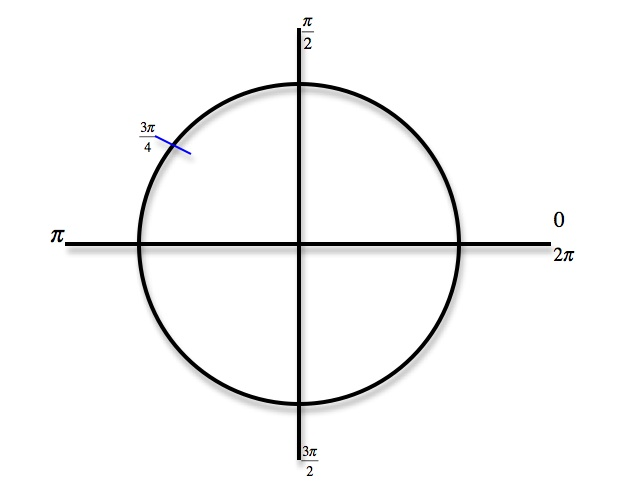• Recall that sine is the y value of the point you just plotted. Now find the angle that has the same sine value of [(3π)/4] between − [(π)/2] and [(π)/2] because − [(π)/2] ≤ arcsin ≤ [(π)/2]
• Draw a line across the y - axis to locate a corresponding point that is between − [(π)/2] and [(π)/2]
•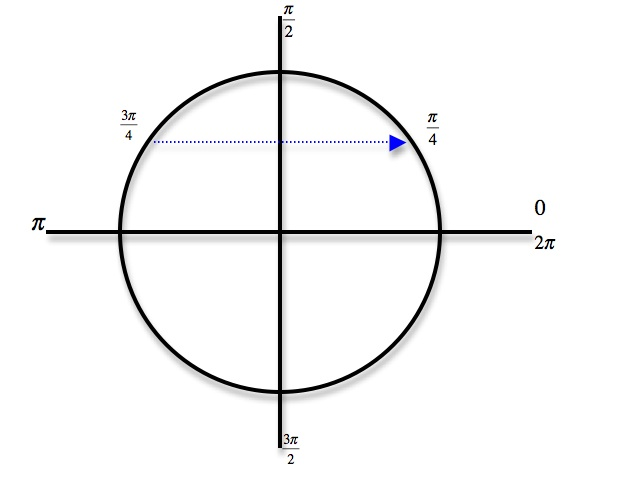arcsin[ sin([(3π)/4]) ] = [(π)/4]
Find arctan[ tan([(2π)/3]) ]
• Plot the point [(2π)/3] on the unit circle. Note: [(2π)/3] = 120°
•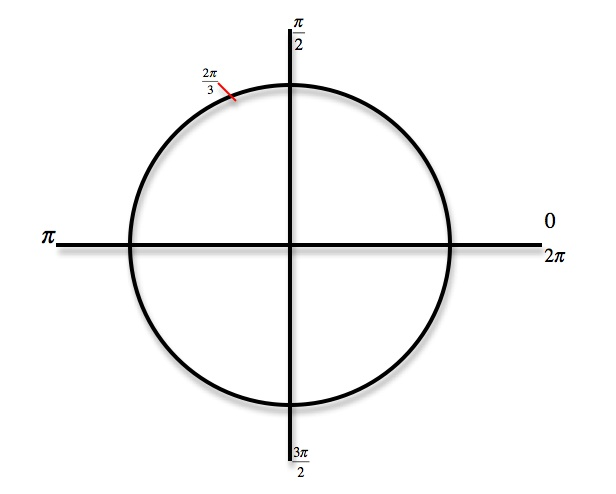• Recall that tangent is [x/y] . Now find the angle that has the same tangent value of [(2π)/3] between − [(π)/2] and [(π)/2] because - [(π)/2] ≤ arctan ≤ [(π)/2]
• Draw a line diagonally across to locate a corresponding point that is between − [(π)/2] and [(π)/2]
•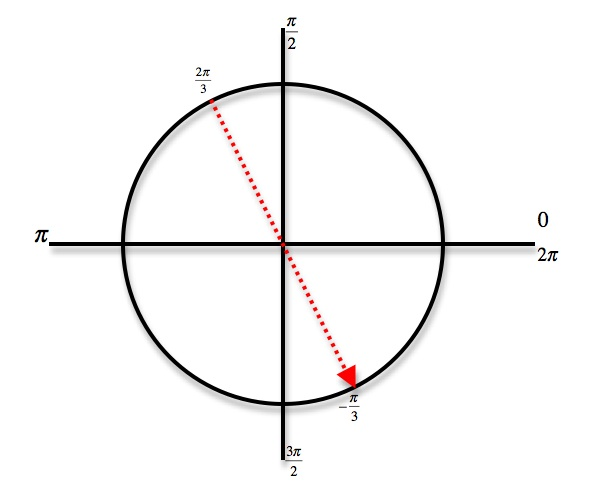arctan [ tan([(2π)/3]) ] = − [(π)/3]
Find arccos[ cos( − [(2π)/3]) ]
• Plot the point − [(2π)/3] on the unit circle. Note: − [(2π)/3] = − 120°
•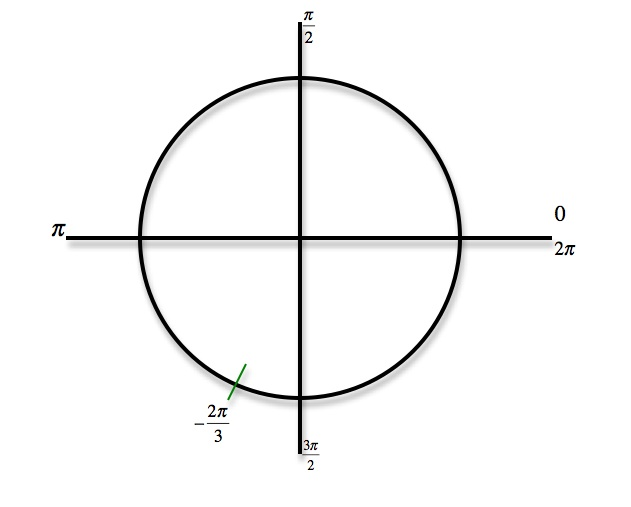• Recall that cosine is the x value of the point you just plotted. Now find the angle that has the same cosine value of − [(2π)/3] between 0 and π because 0 ≤ arccos
• Draw a line across the x - axis to locate a corresponding point that is between 0 and π
•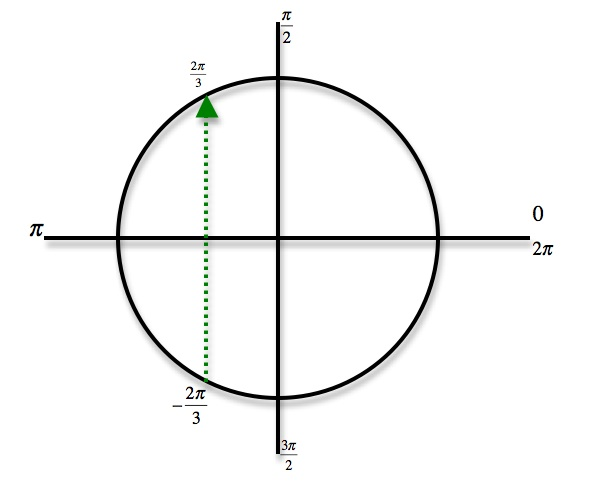arccos[ cos( − [(2π)/3]) ] = [(2π)/3]
Find arcsin[ sin([(5π)/6]) ]
• Plot the point [(5π)/6] on the unit circle. Note: [(5π)/6] = 150°
•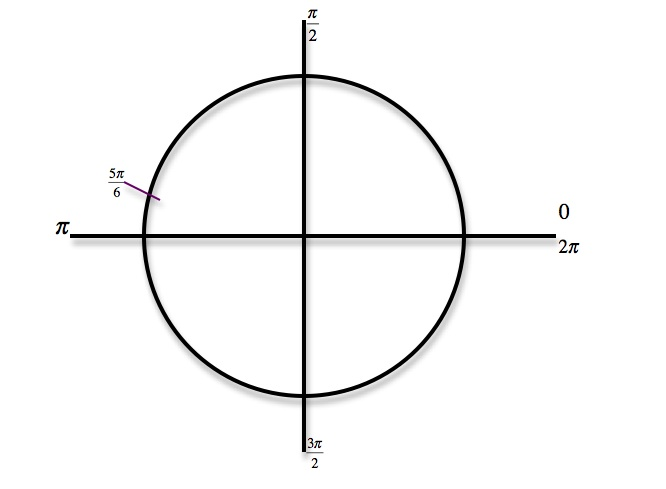• Recall that sine is the y value of the point you just plotted. Now find the angle that has the same sine value of [(5π)/6] between − [(π)/2] and [(π)/2] because - [(π)/2] ≤ arcsin ≤ [(π)/2]
• Draw a line across the y - axis to locate a corresponding point that is between − [(π)/2] and [(π)/2]
•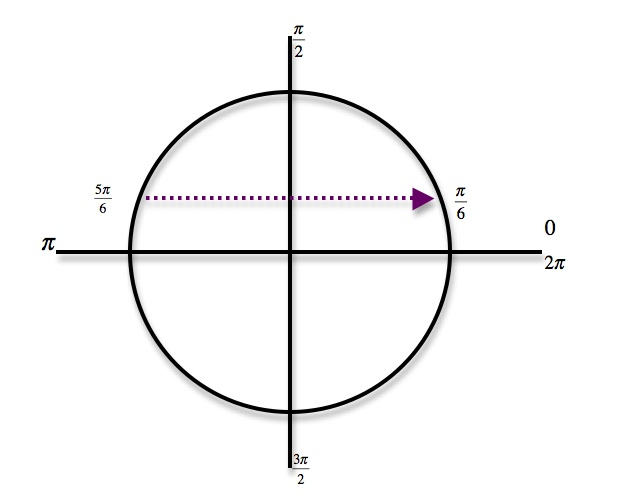arcsin[ sin([(5π)/6]) ] = [(π)/6]
Find arccos[ cos( − [(π)/6]) ]
• Plot the point − [p/6] on the unit circle. Note: − [(π)/6] = − 30°
•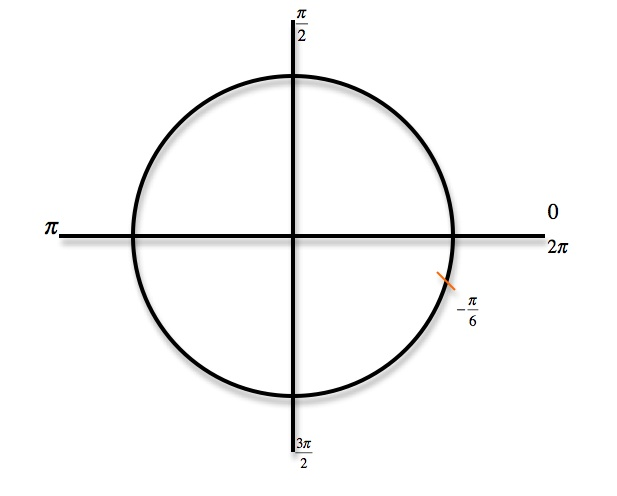• Recall that cosine is the x value of the point you just plotted. Now find the angle that has the same cosine value of − [(π)/6] between 0 and π because 0 ≤ arccos ≤π
• Draw a line across the x - axis to locate a corresponding point that is between 0 and π
•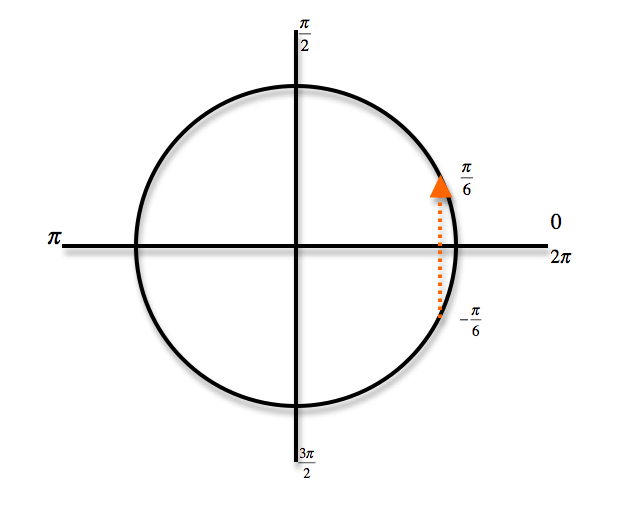arccos[ cos( − [(π)/6]) ] = [(π)/6]
Find arctan[ tan([(4π)/3]) ]
• Plot the point [(4π)/3] on the unit circle. Note: [(4π)/3] = 240°
•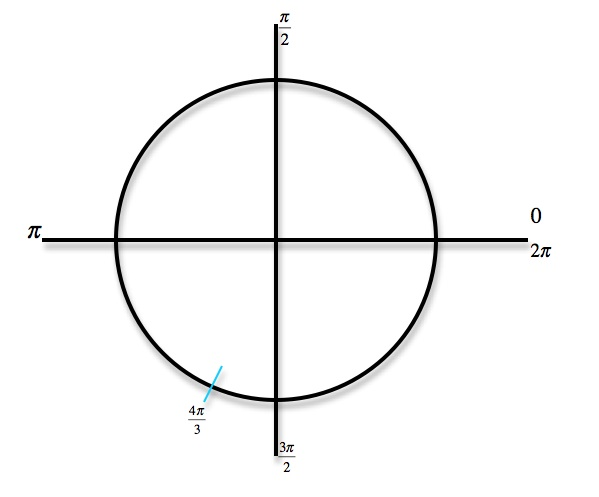• Recall that tangent is [x/y]. Now find the angle that has the same tangent value of [(4π)/3] between − [(π)/2] and [(π)/2] because − [(π)/2] ≤ arctan ≤ [(π)/2]
• Draw a line diagonally across to locate a corresponding point that is between − [(π)/2] and [(π)/2]
•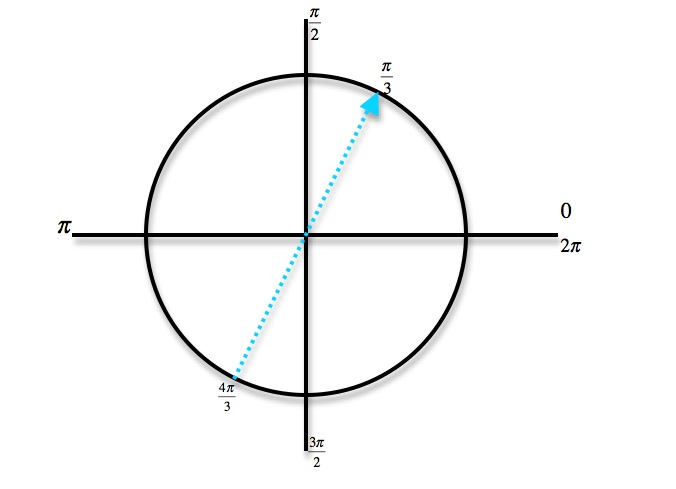arctan[ tan([(4π)/3]) ] = [(π)/3]
Find arcsin[ sin([(5π)/4]) ]
• Plot the point [(5π)/4] on the unit circle. Note: [(5π)/4] = 225°
•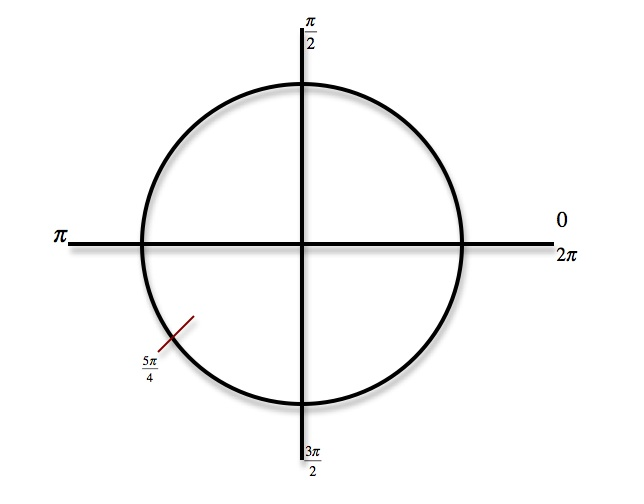• Recall that sine is the y value of the point you just plotted. Now find the angle that has the same sine value of [(5π)/4] between − [(π)/2] and [(π)/2] because - [(π)/2] ≤ arcsin ≤ [(π)/2]
• Draw a line across the y - axis to locate a corresponding point that is between − [(π)/2] and [(π)/2]
•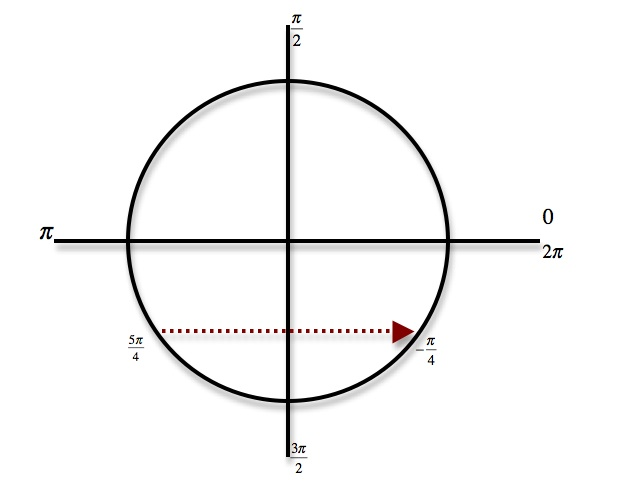arcsin[ sin([(5π)/4]) ] = − [(π)/4]
Find arccos[ cos([(5π)/3]) ]
• Plot the point [(5π)/3] on the unit circle. Note: [(5π)/3] = 300°
•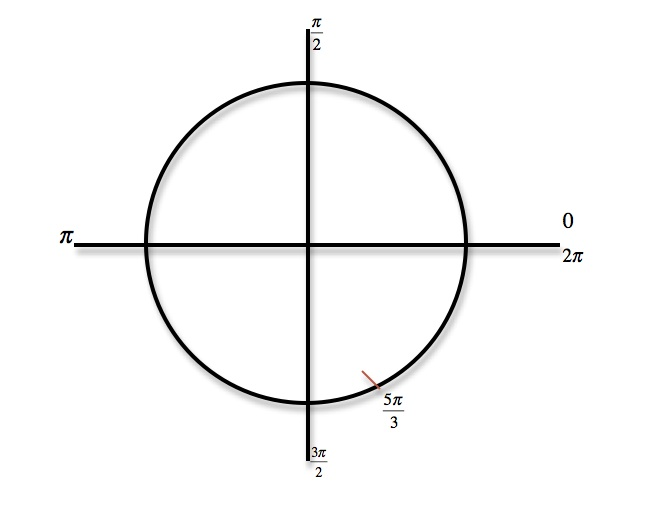• Recall that cosine is the x value of the point you just plotted. Now find the angle that has the same cosine value of [(5π)/3] between 0 and π because 0 ≤ arccos ≤π
• Draw a line across the x - axis to locate a corresponding point that is between 0 and π
•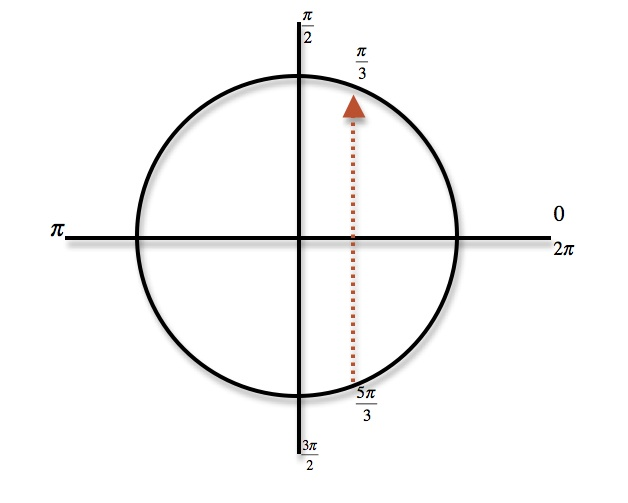arccos[ cos([(5π)/3]) ] = [(π)/3]
Find arctan[ tan( − [(7π)/6]) ]
• Plot the point − [(7π)/6] on the unit circle. Note: − [(7π)/6] = 210°
•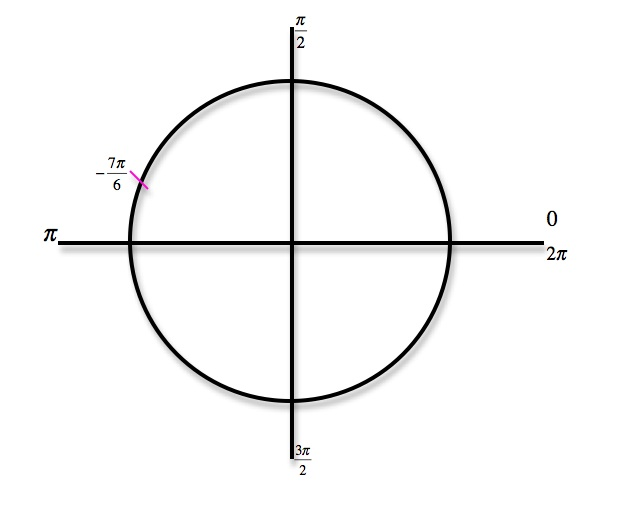• Recall that tangent is [x/y]. Now find the angle that has the same tangent value of − [(7π)/6] between − [(π)/2] and [(π)/2] because - [(π)/2] ≤ arctan ≤ [(π)/2]
• Draw a line diagonally across to locate a corresponding point that is between − [(π)/2] and [(π)/2]
•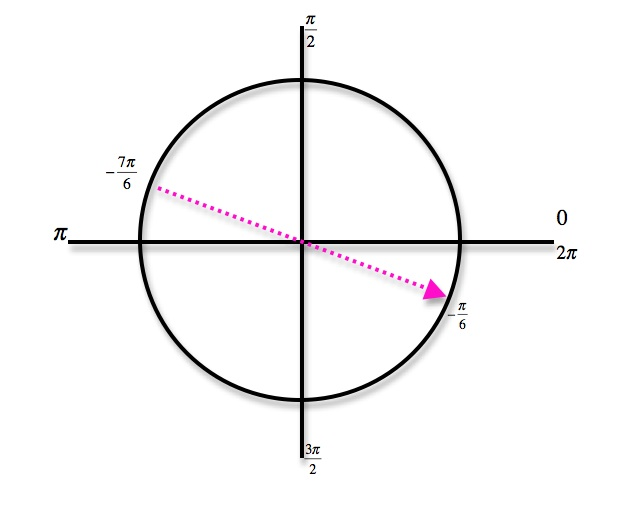arctan[ tan( − [(7π)/6]) ] = − [(π)/6]
Find arcsin[ sin( − [(5π)/6]) ]
• Plot the point − [(5π)/6] on the unit circle. Note: − [(5π)/6] = 150°
•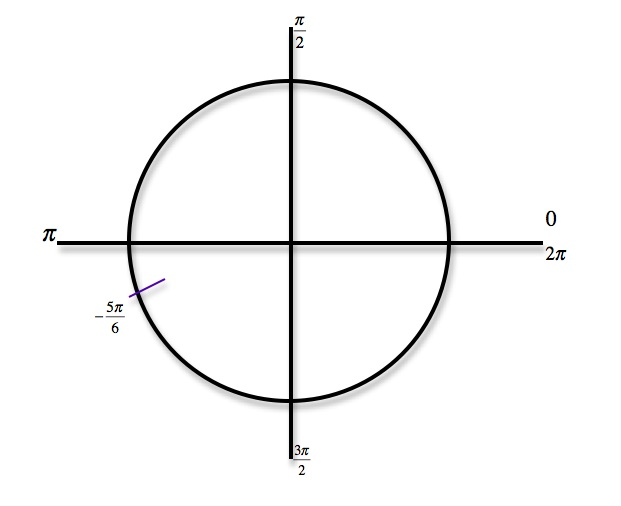• Recall that sine is the y value of the point you just plotted. Now find the angle that has the same sine value of − [(5π)/6] between − [(π)/2] and [(π)/2] because − [(π)/2] ≤ arcsin ≤ [(π)/2]
• Draw a line across the y - axis to locate a corresponding point that is between − [(π)/2] and [(π)/2]
•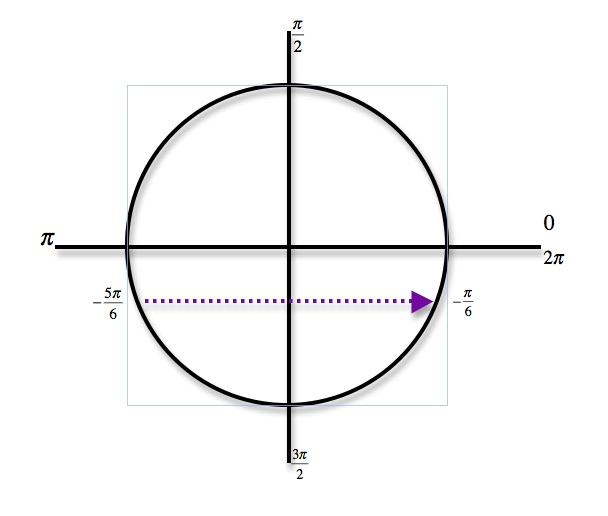arcsin[ sin( − [(5π)/6]) ] = − [(π)/6]

*These practice questions are only helpful when you work on them offline on a piece of paper and then use the solution steps function to check your answer.

### Inverse Trigonometric Functions

Lecture Slides are screen-captured images of important points in the lecture. Students can download and print out these lecture slide images to do practice problems as well as take notes while watching the lecture.

• Intro 0:00
• Arcsine Function 0:24
• Restrictions between -1 and 1
• Arcsine Notation
• Arccosine Function 3:07
• Restrictions between -1 and 1
• Cosine Notation
• Arctangent Function 4:30
• Between -Pi/2 and Pi/2
• Tangent Notation
• Example 1: Domain/Range/Graph of Arcsine 5:45
• Example 2: Arcsin/Arccos/Arctan Values 10:46
• Example 3: Domain/Range/Graph of Arctangent 17:14
• Extra Example 1: Domain/Range/Graph of Arccosine
• Extra Example 2: Arcsin/Arccos/Arctan Values

### Transcription: Inverse Trigonometric Functions

We are trying some more examples of inverse trigonometric functions, now we are asked to identify the domain and range of the arcos function and graph the function.0000

Let me start by graphing the cos function itself.0012

There is pi/2, pi/ -pi/2, 3pi/2, 2pi.0027

What I just graphed there is just cos(theta), I did not graph the arcos function yet and the key thing here is we are going to flip this function around the y=x line.0039

We are going to flip it around the line y=x and we want it to be a function after we flip it.0054

Right now if we flip it the way it is then it would not be a function because it would not pass the vertical line test.0059

We need to cut off just a little piece of the cos function and flip that little piece around and that would give us the arcos function.0067

What we are going to do is we are going to cut off the piece from here at 0 to pi.0077

We are going to cut off this piece, we are going to cut here to make arcos function.0091

The point of doing it like that is if we cut it off that way, we will get something that will pass the vertical line test after we flip it.0111

Let me draw that now, let me draw what we will get when we flip it.0120

If we just take that little piece that we cut off, it started at 0,1.0125

Let me start this one at 1,0, and went down to pi/2,0.0130

Run it up to 0 pi/2 and then it went to pi -1.0141

There is pi on the y axis now and -1 on the x axis and that is our graph of arcos(x).0151

The domain is all the numbers that you can plug into that.0165

It is all (x) would be negative -1, less than or equal to (x), less than or equal to 1.0177

The way you know that is it is all the numbers that can come out of the cos function.0182

Cos always gives you numbers between -1 and 1, those are the numbers that you can plug into arcos.0188

Let me emphasize here that the end points -1 and 1 are included, those are less than and equal to sin.0196

Back when we studied the arctan function, we have to leave the end points out, -pi/2 and pi/2 for arc tan.0209

We left those points out because the function never actually got to those points.0217

Here we include -1 and 1 because the function does get to those points.0225

The range is all (y) with 0 less than (y), less than or equal to pi.0231

0 less than or equal to (y), less than or equal to pi.0245

It is all the (y) values that you could get from the arcos function which in turn corresponds to all the values that go into this little piece of the cos function.0249

It is all about U’s from 0 to pi.0262

If we identified the domain and range and we have got ourselves a graph, we are done with that example.0266

Ok one more example of finding the values of arcsin, arcos, and arctan.0000

I’m going to start by drawing a unit circle.0008

Let me graph the angle that we are going to start with, 4pi/3, there is 0, pi/2, 3pi/2 and 2pi.0032

4pi/3 is down here, there is 4pi/3, it is between pi and 3pi/2.0050

Sin(4pi/3) is the (y) coordinate, the arcsin is always between –pi/2 and pi/2.0061

-pi/2 is at the same place as 3pi/2, we want to find arcsin(sin 4pi/3), we want to find an angle in that range that has the same sin as 4pi/3.0077

Sin is the (y) coordinate, let me keep the (y) coordinate fixed and I will go over and find the point over here, that is –pi/3.0093

Arcsin(sin4pi/3), an angle that has the same sin as 4pi/3 is –pi/3.0110

Make that negative sign a little clear, we have solved the first one.0125

Next one is arcos(cos –pi/4), well there is –pi/4 down there.0131

We want to find an angle that has the same cos but arcos remember is always between 0 and pi, it is always in the first 2 quadrants.0144

We want to find an angle in the first two quadrants that has the same cos as –pi/4.0158

Cos is the (x) value we are keeping the (x) value constant and I’m moving up to find an angle in the first two quadrants, there it is at pi/4.0165

Arcos(cos –pi/4) is pi/4.0181

Finally we have arctan(tan 7pi/6), 7pi/6 is just bigger than pi, there it is down there 7pi/6.0196

We want to find an angle that has the same tan as that.0209

Remember with tan, if we reflect across the origin we will get an angle that has the same sin and cos except they switched from being negative to positive.0213

But they both switched which mean tan(sin/cos) will actually end up the same.0233

This angle is pi/6 will have the same tan as 7pi/6, that is good because arctan is forced to be between –pi/2 and pi/2.0240

We got this angle pi/6 that has the same tan as what we are looking for 7pi/6.0259

The key to solving that problem is to take each one of these angles and find them on the unit circle.0280

Figure out what the range of our function is we are looking for, arctan between –pi/2 and pi/2, arcsin in the same range, arcos little different between 0 and pi.0287

Then we try to find an angle within that range that has the same sin, cos, or tan, as the angle that we started with.0302

That is why I drawn this little dotted lines here on the unit circle, I’m trying to find angles with same cos or same sin, or same tan as what we started with.0313

That what gave us our answers –pi/3, pi/4 and pi/6, those are angles that has the same sin, cos, and tan as the ones we are given.0323

That was our first lecture on inverse trigonometric functions, these are the trigonometry lectures for www.educator.com.0334

Hi, these are the trigonometry lectures for educator.com, and we're here to learn about the inverse trigonometric functions.0000

We've already learned about sine, cosine and tangent, and today we're going to learn about the inverse sine, inverse cosine, and inverse tangent, probably better known as arc sine, arc cosine, and arc tangent.0008

The arc sine function is also known as the inverse sine function, basically, it's kind of the opposite of the sine function.0022

The idea is that you're given a value of x, and you want to find an angle whose sine is that value of x.0030

In order to make this work, sines only occur between -1 and 1, so you have to be given a value of x between -1 and 1.0041

You're going to try to give an angle between -π/2 and π/2.0050

You'll try to give an angle between -π/2 and π/2 that has the given value as a sine.0060

If arcsin(x)=θ, what that really means is that the sin(θ)=x.0078

Now, there's some unfortunate notation in mathematics which is that arcsin is sometimes written as sin to the negative 1 of x.0087

This is very unfortunate because people talk about, for example, sin2x means (sin(x))2.0095

You might think that sin-1(x), would be (sin(x))-1, which would be 1/sin(x).0106

Now, that's not what it means, the sine inverse of x doesn't mean 1/sin(x), it means arcsin(x).0119

This notation really is very ambiguous because this inverse sine notation could mean arcsin(x) or it could mean 1/sin(x), and so this notation is ambiguous because arcsin(x) and 1/sin(x) are not the same.0129

The safest thing to do is to not use this notation sin-1 at all.0151

Let me just say, avoid this notation completely because it is ambiguous, it could be interpreted to mean these two different things that are not equal to each other.0159

Instead, it's probably safer to use the notation arcsin(x), which definitely means inverse sin(x), and cannot be confused.0175

The arccos(x), the arc cosine function is sort of the opposite of the cosine function.0187

You're given a value of x, and you have to find an angle whose cosine is that value of x.0194

Again, the value of x you must be given would have to be between -1 and 1, because those are the only values that come up as answers for cosine.0201

What you try to do is produce an angle between 0 and π, so there's 0, π/2, π.0215

You try to produce an angle between 0 and π that has that value as its cosine.0229

Just like we have with sine, there's the problem of this misleading notation cos-1(x), it could be interpreted as 1/cos(x) or it could be interpreted as arccos(x).0233

The best thing to do is to avoid using this notation completely, cos-1(x) is just misleading, it could be interpreted either way.0247

Try not to use it at all, instead stick to the notation arccos(x).0263

Finally, arc tangent is known as the inverse tangent function.0270

You're given a value of x, and you want to find an angle whose tangent is x.0275

For arc tangent, we're going to try to find the angles between -π/2 and π/2 because that covers all the possible tangents we could get.0282

If arctan(x)=θ, that really means that tan(θ)=x.0297

Just like with sine and cosine, we have this potentially misleading notation, tan-1(x), could be interpreted to mean arctan(x) or 1/tan(x).0302

Those are both reasonable interpretations but they mean two different things.0314

Again, let's try to avoid this notation completely because it could mean two completely different things.0317

We'll just try not to use that at all when we're talking about inverse tangents we'll say arctan instead of tan-1.0337

Let's get started with some examples.0345

First off we have to identify the domain and range of the arc sine function, and then graph the function.0347

Let's start out with the graph of sin(x), because arcsin is really meant to be an inverse to sin(x).0354

Remember that when you're trying to find inverse functions, you take the function and you reflect it across the line y=x.0372

In order for something to be a function, it has to pass the vertical line test.0388

If you draw a vertical line, you shouldn't cross the graph twice.0393

Since we're reflecting across the line y=x, that kind of switches the x's and y's, so we wanted something that will pass the horizontal line test.0397

Let me draw this in red.0408

We don't want to be able to draw a horizontal line and cross the graph twice.0411

As you see, when I've drawn these horizontal lines, we crossed the graph in lots of places.0415

We have a problem when we wanted to find the inverse sine function.0421

The way we solve that is by not using all of the sine graph, we just cut off a piece of the sine graph that will pass the horizontal line test.0425

I'm going to cut off a piece of the sine graph from -π/2 to π/2.0439

If you just look at this portion of the sine graph, you see that it passes the horizontal line test.0448

That means we can take the inverse just at that part of the sine function.0459

Let me draw what that looks like when we reflect it.0465

We're just taking this thick piece here, and I'm going to reflect that across that line y=x.0472

Now, the notations that I had on the y and x axis are going to switch, it goes from now -1 to 1 on the x-axis, and -π/2 to π/2 on the y-axis.0489

I can't keep going with this, I can't draw the rest of the graph because if I do draw any more, I'm going to get something that fails the vertical line test, it won't be a function anymore.0510

This is the entire arcsin function.0521

The domain here, it's all the numbers that you can plug into the arcsin, that's all values of x with -1 less than or equal to x, less than or equal to +1.0532

We can't plug any other values of x into arcsin, and that's really because in the other direction the only values that come out of the sine function are between -1 and 1.0560

The only values that you can plug into the arcsin function are between -1 and 1.0571

The range, the numbers that come out of the arcsin, all values of x between -π/2 and π/2.0580

Let me write that as y because when we think of those are the values coming out of the arcsin function.0596

Those are all the y values you see here and you see that the smallest y-value is -π/2 and the biggest value we see is π/2.0605

Arcsin takes in a number between -1 or 1, gives you a number between -π/2 and π/2 and its graph looks like a sort of chopped of piece of the sine graph.0615

Remember, the reason we had to chop it off is to get a piece of the sine graph that would satisfy the horizontal line test, so that the arcsin graph satisfies the vertical line test and really is a function.0629

In our second example here, we have to find the arcsin of the sin(2π/3) and then some similar values for our cosine and our tangent.0647

The first thing to do here is really to figure out where we are on the unit circle.0663

There's 0, π/2, π, 3π/2 and 2π.0675

Let's figure out where each of this angle is on the unit circle.0685

2π/3 is over here, there's 2π/3.0691

Remember, arcsin is always between -π/2 and π/2.0698

Arcsin is between -π/2 and π/2, so there's -π/2 down here.0712

We want to find an angle between -π/2 and π/2.0717

On the right-hand side that has the same sine as 2π/3.0724

Well, sine is the y-value so we want something that has the same y-value as 2π/3.0729

That angle right there has the same y-coordinate as 2π/3, that's π/3.0741

From the graph, we see that π/3 has the same sine as sin(2π/3).0750

So, arcsin(2π/3) is π/3.0771

It's an angle whose sine is the sin(2π/3).0780

Let's try the next one, -5π/6.0785

-π is over there, so 5π/6 in the negative direction puts you over there, there's -5π/6.0792

We want an angle whose cosine is the same as the cosine(-5π/6).0809

Cosine is the x-value, so we want an angle that has the same x-value as the one we just found.0818

There it is right there, 5π/6.0830

That's between 0 and π, which is the range for the arccos function, it's always between 0 an π.0838

That means that arccos of the cos(-5π/6) is 5π/6, that's an angle between 0 and π that has the right cosine.0850

Finally, we want to find the arctan of tan(3π/4).0875

3π/4 is over there.0882

There's 3π/4.0890

We want to find an angle that has the same tangent as that one.0893

Remember, arctan is forced to be between π/2 and -π/2.0898

We want to find an angle between π/2 and -π/2 that has the same tangent as 3π/4.0909

If we go straight across the origin there, we started with an angle whose cosine is negative and sine is positive.0920

This angle right here has a positive cosine and negative sine, so it'll end up having the same tangent, and that angle is -π/4.0934

That's an angle inside the range we want that has the same tangent.0946

Arctan of tan(3π/4) is -π/4.0952

Those are really quite tricky.0967

What we're being asked to do here in each case is we're given an angle, for example 2π/3.0969

We want to find arcsin of sin(2π/3).0977

We look at the sine of 2π/3, and then we want to find another angle that has that sine but it has to be in the specified range for arcsin.0981

We're really trying to find what's an angle between -π/2 and π/2 whose sine is the same as sin(2π/3).0993

That's why we did this reversal to find the angle π/3 that has the same sine as the sin(2π/3).1003

That was kind of the same process of all three of them, finding angles that have the same cosine as -5π/6, but is in the specified range, finding an angle that has the same tangent as 3π/4 but is in the specified range.1017

Our third example here, we're asked to identify the domain and range of the arctan function, and graph the function.1037

Remember when we graphed arcsin, we started out with a graph of sine, so let me start out here with a graph of tangent.1044

I'll graph it in blue.1054

Has asymptotes of π/2, and then it starts repeating itself.1057

It has period π.1065

This is 0, π/2, -π/2, and that's π, 3π/2.1084

What I graphed in blue there is tan(θ).1096

Now, I want to take this graph and I want to flip it around the line y=x.1102

Let me graph the line y=x.1107

I want to get something that it will be a function, it has to pass the vertical line test after I flip it.1112

That means it has to pass the horizontal line test before I flip it.1120

Just like with sine, the tangent function fails the horizontal line test badly.1124

You can draw a horizontal lines and intersect it in lots of places.1132

Just like with sine, we're going to cut off part of the tangent function and use that to form the arctan function.1137

We're going to cut off just one of these curves.1143

I'll cut it off these asymptotes at -π/2 and π/2.1151

I'll just take this part of the tangent function and I'll flip it around to make the arctan function.1156

I'll do this in red.1171

Arctan(x).1179

I'm going to flip this around just that one main branch of the tangent function.1181

Since the tangent function had vertical asymptotes, the arctan function is going to have horizontal asymptotes at -π/2 and π/2.1192

Those are my asymptotes right there at -π/2 and π/2.1210

We're also asked to identify the domain and range.1216

The domain means what numbers can we plug in to arctan(x).1219

Domain is all values of x because we can plug any number in on the x-axis here and we'll get an arctan value.1228

-infinity less than x less than infinity.1243

The range is all x with, okay, let me write this as y because these are values on the y-axis.1248

All y with -π/2 less than y less than π/2.1264

Let me emphasize here that π/2 and -π/2 are not included, π/2 themselves are not in the range.1269

These inequalities, they're not less than or equal to they're strictly less than.1291

The reason for that is that the arctan function never quite gets to -π/2 or π/2, it goes down close -π/2 and it goes up close to π/2 but it never quite gets there.1297

The reason for that, if you kind of look back at the tangent function, was that these asymptotes never quite get to -π/2 or π/2.1312

You can't take the tangent of -π/2 or π/2, because you're trying to divide by zero back in the tangent function.1323

To understand this problem really, you have to remember what the graph of tan(θ) looks like.1333

Then you take its one main branch and you flip it over, and you get the graph of arctan(x), and the domain and range just come back to any things in the domain.1339

The range can go from π/2 to -π/2, but you don't include those end points.1354

The asymptotes are the horizontal lines at -π/2 and π/2.1362

OR

### Start Learning Now

Our free lessons will get you started (Adobe Flash® required).